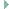arduino_board.h File Reference

Board specific configuration for the Arduino API. More...

## Detailed Description

Board specific configuration for the Arduino API.

Definition in file arduino_board.h.

`#include "periph/gpio.h"`
`#include "periph/adc.h"`
`#include "periph/pwm.h"`Include dependency graph for arduino_board.h:

Go to the source code of this file.

 #define ARDUINO_LED   (13) The builtin LED. #define ARDUINO_UART_DEV   UART_DEV(0) On-board serial port mapping. #define ARDUINO_PWM_FREQU   (732U) PWM frequency. static const gpio_t arduino_pinmap [] Look-up table for the Arduino's digital pins. static const adc_t arduino_analog_map [] Look-up table for the Arduino's analog pins. More... static const arduino_pwm_t arduino_pwm_list [] List of PWM GPIO mappings. More...

## ◆ arduino_analog_map

static
Initial value:
= {
}

Look-up table for the Arduino's analog pins.

Definition at line 131 of file arduino_board.h.

## ◆ arduino_pwm_list

 const arduino_pwm_t arduino_pwm_list[]
static
Initial value:
= {
{ .pin = 20, .dev = PWM_DEV(0), .chan = 0 },
{ .pin = 29, .dev = PWM_DEV(0), .chan = 1 },
{ .pin = 11, .dev = PWM_DEV(1), .chan = 0 },
{ .pin = 13, .dev = PWM_DEV(1), .chan = 1 },
{ .pin = 14, .dev = PWM_DEV(1), .chan = 2 },
}
#define PWM_DEV(x)
Default PWM access macro.
Definition: pwm.h:78

List of PWM GPIO mappings.

Definition at line 157 of file arduino_board.h.